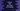# Python program to find the power of a number using Anonymous function## Python program to find the power of a number using Anonymous function:

In this post, we will learn how to find the power of a number in range by using an anonymous function. This program will take one number as input from the user, it will find all powers for that number from 0 to 10 and print one table, i.e. the first line will print power to 0, second line will print power to 1 etc.

We will create one list of all power values for that number by using a anonymous function. Then, it will iterate through the values and print out the powers.

Anonymous or lambda function can be used without using a name and it makes the code simpler.

With this program, you will learn how to use anonymous or lambda function in Python.

### Python program:

Below is the complete python program:

``````number = int(input('Enter a number: '))

list_power = list(map(lambda x: number ** x, range(11)))

for i in range(11):
print('{} raised to power {} is {}'.format(number, i, list_power[i]))``````

Here,

• The program is taking one number as input from the user and keeping it in the variable number
• list_power is a list created by using a lambda function. This function creates a list of powers for the number.
• The last for loop prints the table.

If you run this program, it will print output as like below:

``````Enter a number: 10
10 raised to power 0 is 1
10 raised to power 1 is 10
10 raised to power 2 is 100
10 raised to power 3 is 1000
10 raised to power 4 is 10000
10 raised to power 5 is 100000
10 raised to power 6 is 1000000
10 raised to power 7 is 10000000
10 raised to power 8 is 100000000
10 raised to power 9 is 1000000000
10 raised to power 10 is 10000000000

Enter a number: 2
2 raised to power 0 is 1
2 raised to power 1 is 2
2 raised to power 2 is 4
2 raised to power 3 is 8
2 raised to power 4 is 16
2 raised to power 5 is 32
2 raised to power 6 is 64
2 raised to power 7 is 128
2 raised to power 8 is 256
2 raised to power 9 is 512
2 raised to power 10 is 1024``````